# Polyglot Data Science with IPython & friends¶

A demonstration of how to use Python, Julia, Fortran and R cooperatively to analyze data, in the same process.

This is supported by the IPython kernel and a few extensions that take advantage of IPython's magic system to provide low-level integration between Python and other languages.

See the companion notebook for data preparation and setup.

In :
%matplotlib inline
import matplotlib.pyplot as plt
import numpy as np
import pandas as pd
import seaborn as sns

In :
plt.style.use("seaborn")
plt.rcParams["figure.figsize"] = (10, 6)
sns.set_context("talk", font_scale=1.4)


Let's begin by reading our dataset and having a quick look:

In :
data = pd.read_csv('data.csv')
print(data.shape)

(300, 2)

Out:
x y
0 0.000000 0.094287
1 0.021014 -0.216828
2 0.042028 0.329982

Ah, it looks like we have a quantitative dataset with $(x,y)$ pairs - a scatterplot is a decent starting point to get a feel for these data:

In :
data.plot.scatter(x='x', y='y');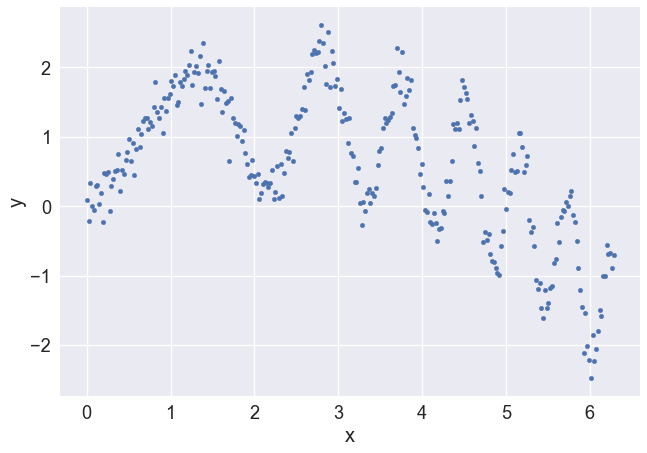Mmh, what to do?

Let's try to build a simple linear model for these data, with some features we'll extract from the data. There's probably:

• a linear dependence
• something that looks broadly non-linear, let's say quadratic, to keep things simple
• and maybe an oscillatorty term: but it looks a bit like a chirp, not a simple sinusoid. Again, simplest non-linear choice: quadratic frequency dependency.

In summary, let's try

$$y \sim \theta_1 x + \theta_2 x^2 + \theta_3 \sin(x^2)$$

## Oh boy! Complicated data, Julia to the rescue...¶

Maybe Julia can help us efficiently compute that nasty non-linear feature, $x^2$?

In :
%load_ext julia.magic

Initializing Julia interpreter. This may take some time...

In :
jxsq = %julia xsq(x) = x.^2  # Simplest way to define a function in Julia


We've defined the function xsq in Julia, and in Python we have it available as jxsq, which we can call as a normal Python function:

In :
x = data['x']
f2 = jxsq(x)
x.shape == f2.shape  # our feature should have the same shape as our data

Out:
True

# More complicated features, we need performance...¶

## Fortran can help us!¶

Let's use this oldie but goodie to assist in the task of estimating our next non-linear feature, $\sin(x^2)$:

In :
%load_ext fortranmagic

/Users/bussonniermatthias/anaconda/lib/python3.6/site-packages/fortranmagic.py:147: UserWarning: get_ipython_cache_dir has moved to the IPython.paths module since IPython 4.0.
self._lib_dir = os.path.join(get_ipython_cache_dir(), 'fortran')

In :
%%fortran
subroutine sinx2(x, y, n)
real, intent(in), dimension(n) :: x
real, intent(out), dimension(n) :: y
!intent(hide) :: n
y = sin(x**2)
end subroutine sinx2


Now, sinx2 can be used as a plain Python function too:

In :
#f3 = sinx2(x)
f3 = np.sin(x**2)

f3.shape == x.shape  # same sanity check

Out:
True

# Statistical modeling? Maybe R can do that??¶

We now have our data y and our features $x$, $f_2 = x^2$ and $f_3 = \sin(x^2)$. This is a classic linear modeling problem, and R is awesome at fitting those!

Let's put our features together in a nice design matrix and load up R:

In :
A = np.column_stack([x, f2, f3])
A.shape

Out:
(300, 3)
In :
%load_ext rpy2.ipython
y = data['y']


In R, this can be written as a linear model lm(y ~ 0 + A).

Note that we'll ask for the fit coefficients fitc to keep moving forward:

In :
%%R -i y,A -o fitc

ylm = lm(y ~ 0 + A)
fitc = coef(ylm)
print(summary(ylm))
par(mfrow=c(2,2))
plot(ylm)

Call:
lm(formula = y ~ 0 + A)

Residuals:
Min      1Q  Median      3Q     Max
-0.7186 -0.1182  0.0090  0.1275  0.5450

Coefficients:
Estimate Std. Error t value Pr(>|t|)
A1  1.000707   0.012517   79.95   <2e-16 ***
A2 -0.199631   0.002566  -77.81   <2e-16 ***
A3  1.015147   0.016646   60.98   <2e-16 ***
---
Signif. codes:  0 ‘***’ 0.001 ‘**’ 0.01 ‘*’ 0.05 ‘.’ 0.1 ‘ ’ 1

Residual standard error: 0.1958 on 297 degrees of freedom
Multiple R-squared:  0.9741,	Adjusted R-squared:  0.9739
F-statistic:  3730 on 3 and 297 DF,  p-value: < 2.2e-16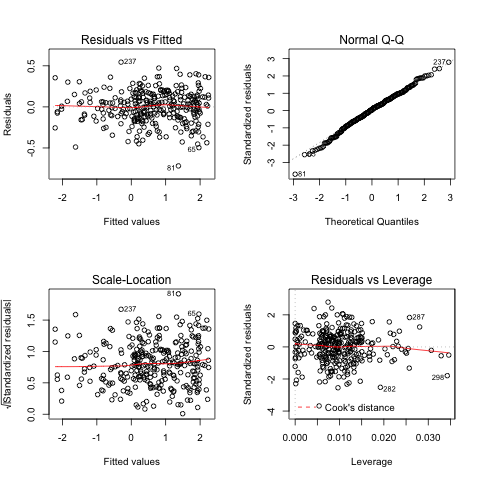# Back to Python to look at the results!¶

R gave us our fit coefficient vector fitc, we can now proceed using it:

In :
fitc

Out:
array([ 1.00070744, -0.19963147,  1.01514731])

We construct our fitted model and visualize our results:

In :
yfit = A @ fitc

In :
plt.plot(x, y, 'o', label='data')
plt.plot(x, yfit, label='fit', color='orange', lw=4)
plt.title('Julia, Python and R working in Jupyter')
plt.legend();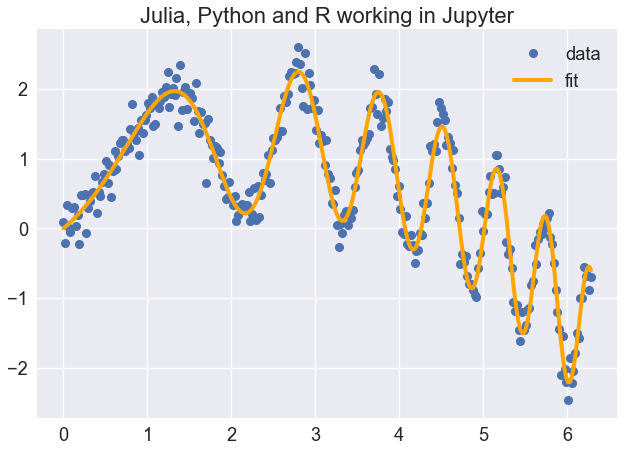# Bonus¶

## Cython, the happy child of C and Python¶

In :
def f(x):
return x**2-x

def integrate_f(a, b, N):
s = 0; dx = (b-a)/N
for i in range(N):
s += f(a+i*dx)
return s * dx

In :
%load_ext Cython

In :
%%cython -a
cdef double fcy(double x) except? -2:
return x**2-x

def integrate_fcy(double a, double b, int N):
cdef int i
cdef double s, dx
s = 0; dx = (b-a)/N
for i in range(N):
s += fcy(a+i*dx)
return s * dx

Out:
Cython: _cython_magic_8ba296f194edf8ff3bbb003f9da689f4.pyx

Generated by Cython 0.27.1

Yellow lines hint at Python interaction.
Click on a line that starts with a "+" to see the C code that Cython generated for it.

+01: cdef double fcy(double x) except? -2:
static double __pyx_f_46_cython_magic_8ba296f194edf8ff3bbb003f9da689f4_fcy(double __pyx_v_x) {
double __pyx_r;
__Pyx_RefNannyDeclarations
__Pyx_RefNannySetupContext("fcy", 0);
/* … */
/* function exit code */
__pyx_L0:;
__Pyx_RefNannyFinishContext();
return __pyx_r;
}

+02:     return x**2-x
  __pyx_r = (pow(__pyx_v_x, 2.0) - __pyx_v_x);
goto __pyx_L0;

 03:
+04: def integrate_fcy(double a, double b, int N):
/* Python wrapper */
static PyObject *__pyx_pw_46_cython_magic_8ba296f194edf8ff3bbb003f9da689f4_1integrate_fcy(PyObject *__pyx_self, PyObject *__pyx_args, PyObject *__pyx_kwds); /*proto*/
static PyMethodDef __pyx_mdef_46_cython_magic_8ba296f194edf8ff3bbb003f9da689f4_1integrate_fcy = {"integrate_fcy", (PyCFunction)__pyx_pw_46_cython_magic_8ba296f194edf8ff3bbb003f9da689f4_1integrate_fcy, METH_VARARGS|METH_KEYWORDS, 0};
static PyObject *__pyx_pw_46_cython_magic_8ba296f194edf8ff3bbb003f9da689f4_1integrate_fcy(PyObject *__pyx_self, PyObject *__pyx_args, PyObject *__pyx_kwds) {
double __pyx_v_a;
double __pyx_v_b;
int __pyx_v_N;
PyObject *__pyx_r = 0;
__Pyx_RefNannyDeclarations
__Pyx_RefNannySetupContext("integrate_fcy (wrapper)", 0);
{
static PyObject **__pyx_pyargnames[] = {&__pyx_n_s_a,&__pyx_n_s_b,&__pyx_n_s_N,0};
PyObject* values = {0,0,0};
if (unlikely(__pyx_kwds)) {
Py_ssize_t kw_args;
const Py_ssize_t pos_args = PyTuple_GET_SIZE(__pyx_args);
switch (pos_args) {
case  3: values = PyTuple_GET_ITEM(__pyx_args, 2);
CYTHON_FALLTHROUGH;
case  2: values = PyTuple_GET_ITEM(__pyx_args, 1);
CYTHON_FALLTHROUGH;
case  1: values = PyTuple_GET_ITEM(__pyx_args, 0);
CYTHON_FALLTHROUGH;
case  0: break;
default: goto __pyx_L5_argtuple_error;
}
kw_args = PyDict_Size(__pyx_kwds);
switch (pos_args) {
case  0:
if (likely((values = PyDict_GetItem(__pyx_kwds, __pyx_n_s_a)) != 0)) kw_args--;
else goto __pyx_L5_argtuple_error;
CYTHON_FALLTHROUGH;
case  1:
if (likely((values = PyDict_GetItem(__pyx_kwds, __pyx_n_s_b)) != 0)) kw_args--;
else {
__Pyx_RaiseArgtupleInvalid("integrate_fcy", 1, 3, 3, 1); __PYX_ERR(0, 4, __pyx_L3_error)
}
CYTHON_FALLTHROUGH;
case  2:
if (likely((values = PyDict_GetItem(__pyx_kwds, __pyx_n_s_N)) != 0)) kw_args--;
else {
__Pyx_RaiseArgtupleInvalid("integrate_fcy", 1, 3, 3, 2); __PYX_ERR(0, 4, __pyx_L3_error)
}
}
if (unlikely(kw_args > 0)) {
if (unlikely(__Pyx_ParseOptionalKeywords(__pyx_kwds, __pyx_pyargnames, 0, values, pos_args, "integrate_fcy") < 0)) __PYX_ERR(0, 4, __pyx_L3_error)
}
} else if (PyTuple_GET_SIZE(__pyx_args) != 3) {
goto __pyx_L5_argtuple_error;
} else {
values = PyTuple_GET_ITEM(__pyx_args, 0);
values = PyTuple_GET_ITEM(__pyx_args, 1);
values = PyTuple_GET_ITEM(__pyx_args, 2);
}
__pyx_v_a = __pyx_PyFloat_AsDouble(values); if (unlikely((__pyx_v_a == (double)-1) && PyErr_Occurred())) __PYX_ERR(0, 4, __pyx_L3_error)
__pyx_v_b = __pyx_PyFloat_AsDouble(values); if (unlikely((__pyx_v_b == (double)-1) && PyErr_Occurred())) __PYX_ERR(0, 4, __pyx_L3_error)
__pyx_v_N = __Pyx_PyInt_As_int(values); if (unlikely((__pyx_v_N == (int)-1) && PyErr_Occurred())) __PYX_ERR(0, 4, __pyx_L3_error)
}
goto __pyx_L4_argument_unpacking_done;
__pyx_L5_argtuple_error:;
__Pyx_RaiseArgtupleInvalid("integrate_fcy", 1, 3, 3, PyTuple_GET_SIZE(__pyx_args)); __PYX_ERR(0, 4, __pyx_L3_error)
__pyx_L3_error:;
__Pyx_RefNannyFinishContext();
return NULL;
__pyx_L4_argument_unpacking_done:;
__pyx_r = __pyx_pf_46_cython_magic_8ba296f194edf8ff3bbb003f9da689f4_integrate_fcy(__pyx_self, __pyx_v_a, __pyx_v_b, __pyx_v_N);

/* function exit code */
__Pyx_RefNannyFinishContext();
return __pyx_r;
}

static PyObject *__pyx_pf_46_cython_magic_8ba296f194edf8ff3bbb003f9da689f4_integrate_fcy(CYTHON_UNUSED PyObject *__pyx_self, double __pyx_v_a, double __pyx_v_b, int __pyx_v_N) {
int __pyx_v_i;
double __pyx_v_s;
double __pyx_v_dx;
PyObject *__pyx_r = NULL;
__Pyx_RefNannyDeclarations
__Pyx_RefNannySetupContext("integrate_fcy", 0);
/* … */
/* function exit code */
__pyx_L1_error:;
__Pyx_XDECREF(__pyx_t_4);
__pyx_r = NULL;
__pyx_L0:;
__Pyx_XGIVEREF(__pyx_r);
__Pyx_RefNannyFinishContext();
return __pyx_r;
}
/* … */
__pyx_tuple_ = PyTuple_Pack(6, __pyx_n_s_a, __pyx_n_s_b, __pyx_n_s_N, __pyx_n_s_i, __pyx_n_s_s, __pyx_n_s_dx); if (unlikely(!__pyx_tuple_)) __PYX_ERR(0, 4, __pyx_L1_error)
__Pyx_GOTREF(__pyx_tuple_);
__Pyx_GIVEREF(__pyx_tuple_);
/* … */
__pyx_t_1 = PyCFunction_NewEx(&__pyx_mdef_46_cython_magic_8ba296f194edf8ff3bbb003f9da689f4_1integrate_fcy, NULL, __pyx_n_s_cython_magic_8ba296f194edf8ff3b); if (unlikely(!__pyx_t_1)) __PYX_ERR(0, 4, __pyx_L1_error)
__Pyx_GOTREF(__pyx_t_1);
if (PyDict_SetItem(__pyx_d, __pyx_n_s_integrate_fcy, __pyx_t_1) < 0) __PYX_ERR(0, 4, __pyx_L1_error)
__Pyx_DECREF(__pyx_t_1); __pyx_t_1 = 0;

 05:     cdef int i
 06:     cdef double s, dx
+07:     s = 0; dx = (b-a)/N
  __pyx_v_s = 0.0;
__pyx_t_1 = (__pyx_v_b - __pyx_v_a);
if (unlikely(__pyx_v_N == 0)) {
PyErr_SetString(PyExc_ZeroDivisionError, "float division");
__PYX_ERR(0, 7, __pyx_L1_error)
}
__pyx_v_dx = (__pyx_t_1 / ((double)__pyx_v_N));

+08:     for i in range(N):
  __pyx_t_2 = __pyx_v_N;
for (__pyx_t_3 = 0; __pyx_t_3 < __pyx_t_2; __pyx_t_3+=1) {
__pyx_v_i = __pyx_t_3;

+09:         s += fcy(a+i*dx)
    __pyx_t_1 = __pyx_f_46_cython_magic_8ba296f194edf8ff3bbb003f9da689f4_fcy((__pyx_v_a + (__pyx_v_i * __pyx_v_dx))); if (unlikely(__pyx_t_1 == ((double)-2.0) && PyErr_Occurred())) __PYX_ERR(0, 9, __pyx_L1_error)
__pyx_v_s = (__pyx_v_s + __pyx_t_1);
}

+10:     return s * dx
  __Pyx_XDECREF(__pyx_r);
__pyx_t_4 = PyFloat_FromDouble((__pyx_v_s * __pyx_v_dx)); if (unlikely(!__pyx_t_4)) __PYX_ERR(0, 10, __pyx_L1_error)
__Pyx_GOTREF(__pyx_t_4);
__pyx_r = __pyx_t_4;
__pyx_t_4 = 0;
goto __pyx_L0;

In :
tpy = %timeit -o -n 1000 integrate_f(0, 1, 100)
tcy = %timeit -o -n 1000 integrate_fcy(0, 1, 100)

35.9 µs ± 3.32 µs per loop (mean ± std. dev. of 7 runs, 1000 loops each)
343 ns ± 57.9 ns per loop (mean ± std. dev. of 7 runs, 1000 loops each)

In :
tpy.best/tcy.best

Out:
99.14938925050228

## The Julia interoperability is pretty neat¶

In :
%julia @pyimport numpy as np
%julia @pyimport matplotlib.pyplot as plt

In :
%%julia
# Note how we mix numpy and julia:
t = linspace(0,2*pi,1000); # use the julia linspace
s = sin(3*t + 4*np.cos(2*t)); # use the numpy cosine and julia sine
fig = plt.gcf()
plt.plot(t, s, color="red", linewidth=2.0, linestyle="--")

Out:
[<matplotlib.lines.Line2D at 0x1344b0d68>]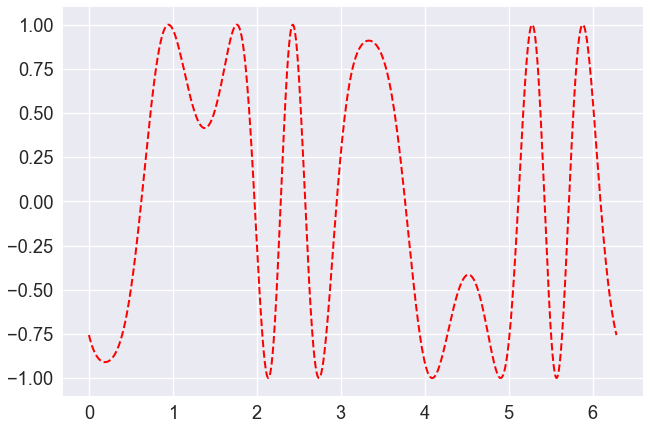In :
fig = %julia fig
fig

Out: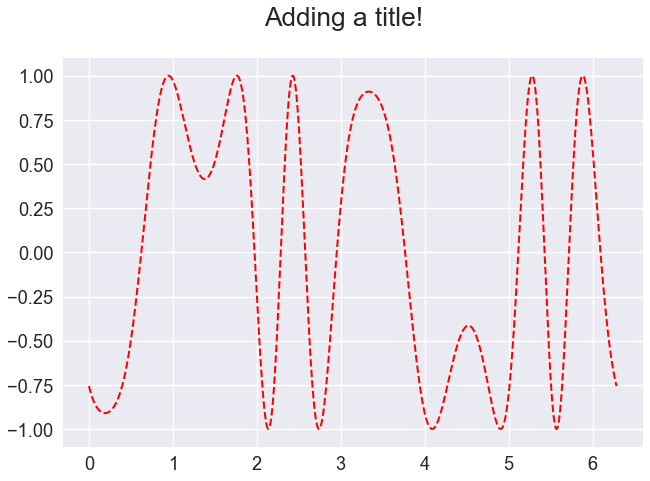## And you can really use Fortran as an interactive language¶

In :
%%fortran
subroutine f1(x, y, n)
real, intent(in), dimension(n) :: x
real, intent(out), dimension(n) :: y
!intent(hide) :: n
y = sin(xx**2) - cos(x)
end subroutine f1

In :
t = np.linspace(0,2* np.pi, 1000)
plt.plot(f1(t));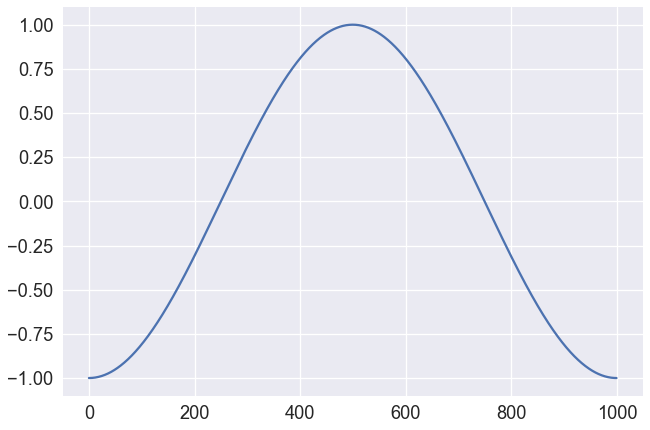# Bash, Perl, Ruby, etc...¶

In :
%%bash
echo $HOME  /Users/bussonniermatthias  In : %%perl @months = ("Jan", "Feb", "Mar"); print($months)

Feb